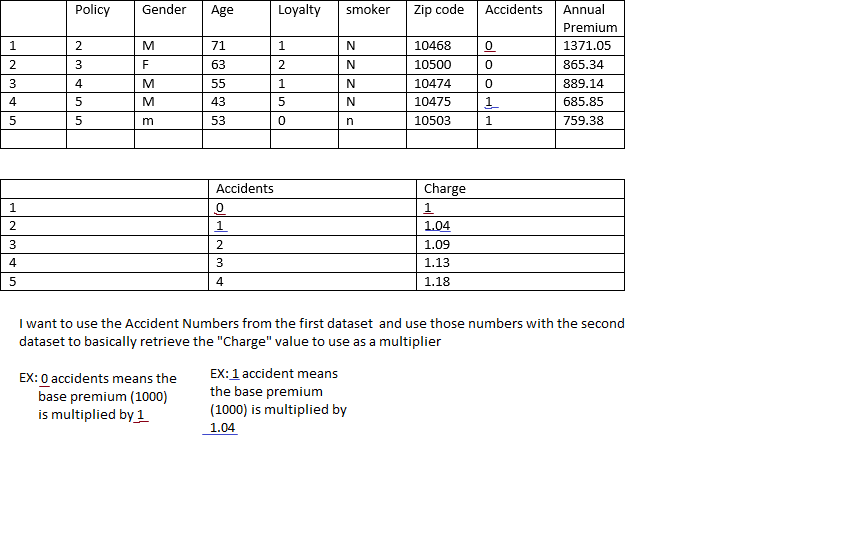## Using data from two datasets for an equation.

I have multiple different data sets, and I am trying to use two at a time for calculations. For instance:

I have a dataset with approximately 90,000 rows, each row has columns: policy , age, gender, loyalty (years with company), smoker, zip code, accidents, premium.

I have another dataset with 6 rows. each row has columns: accidents, Charge.

What I am trying to do is take the accidents from the first dataset for each row and reference that number of accidents with the same number in the second dataset under the accidents column, and then use what is in the charge column for that many accidents (by row) as a multiplier.

I have spent a few hours trying different things and haven't really come up with any solutions.

EDIT: I have uploaded a visual I quickly made to make it easier to understand what I am trying to accomplish6 REPLIES 6

## Re: Using data from two datasets for an equation.

This is a lookup, there are quite a few posts on here about the most efficient way. I suggest looking into proc format and a left join.

Here's a paper that lists several other options:
http://www2.sas.com/proceedings/forum2008/095-2008.pdf

## Re: Using data from two datasets for an equation.

I'm not having any luck with that info. All I can figure out how to do with a lookup is take an individual policy and perform my desired actions, I need to create a new table with every value from one dataset multiplied by its corresponding value from the other dataset

## Re: Using data from two datasets for an equation.

Hi,

```proc sql;
create table WANT as
select  A.*,
B.CHARGE,
from    WORK.HAVE A
left join WORK.ACCIDENTS
on      A.ACCIDENTS=B.ACCIDENTS;
quit;```

## Re: Using data from two datasets for an equation.

Thanks, I'm going to bed now But I will work with this in the morning!

## Re: Using data from two datasets for an equation.

data one;
input policy gender\$ age loyalty smoker\$ zipcode accidents annual_premium;
cards;
2 M 71 1 N 10468 0 1371.05
3 F 63 2 M 10500 1 865.34
;
run;

data two;
input accidents charge;
cards;
0 1
1 1.04
;
run;

data want;
merge one (in=a)
two (in=b);
by accidents;
if a and b;